Research papers and code for "recurrent neural network":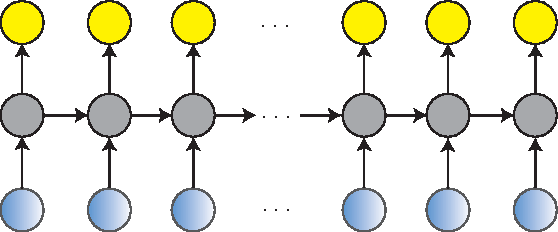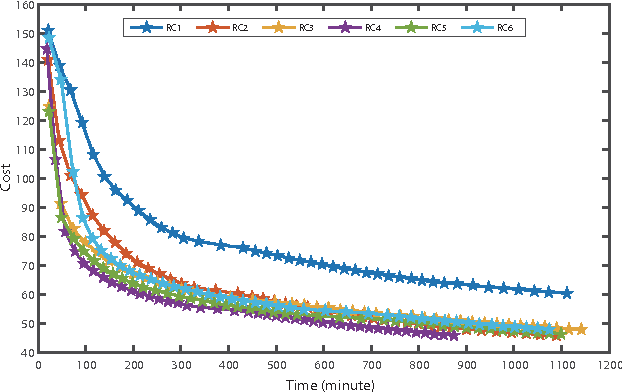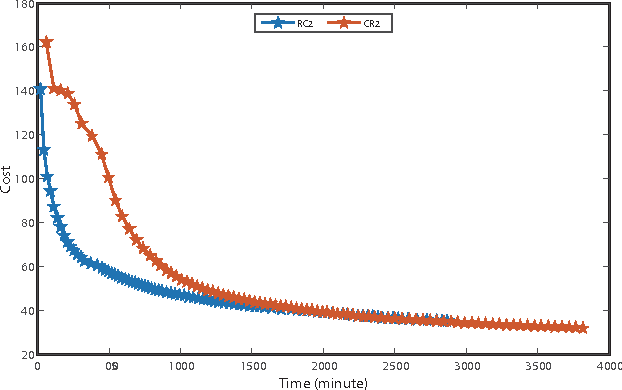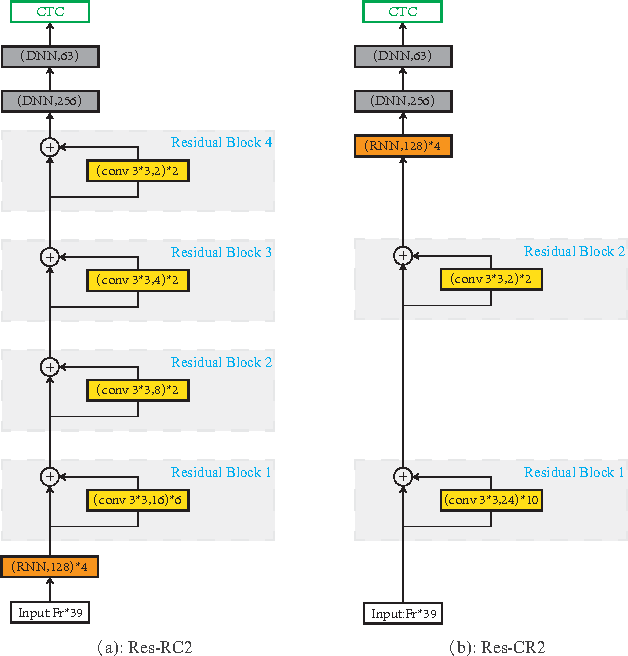A deep learning approach has been widely applied in sequence modeling problems. In terms of automatic speech recognition (ASR), its performance has significantly been improved by increasing large speech corpus and deeper neural network. Especially, recurrent neural network and deep convolutional neural network have been applied in ASR successfully. Given the arising problem of training speed, we build a novel deep recurrent convolutional network for acoustic modeling and then apply deep residual learning to it. Our experiments show that it has not only faster convergence speed but better recognition accuracy over traditional deep convolutional recurrent network. In the experiments, we compare the convergence speed of our novel deep recurrent convolutional networks and traditional deep convolutional recurrent networks. With faster convergence speed, our novel deep recurrent convolutional networks can reach the comparable performance. We further show that applying deep residual learning can boost the convergence speed of our novel deep recurret convolutional networks. Finally, we evaluate all our experimental networks by phoneme error rate (PER) with our proposed bidirectional statistical n-gram language model. Our evaluation results show that our newly proposed deep recurrent convolutional network applied with deep residual learning can reach the best PER of 17.33\% with the fastest convergence speed on TIMIT database. The outstanding performance of our novel deep recurrent convolutional neural network with deep residual learning indicates that it can be potentially adopted in other sequential problems.

* 11 pages, 13 figures
Click to Read Paper and Get Code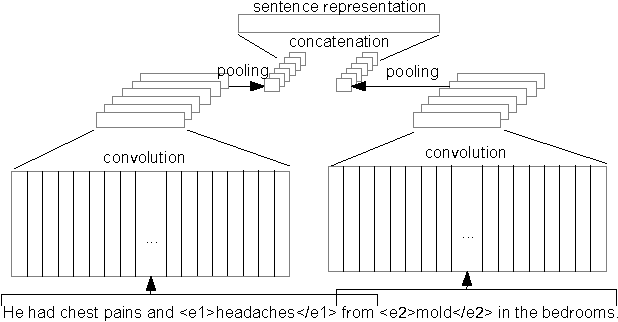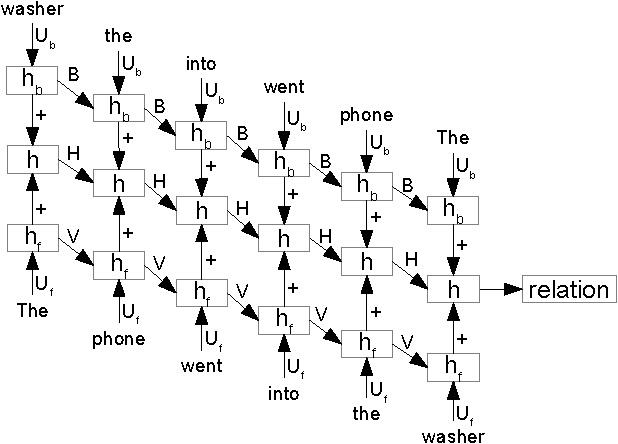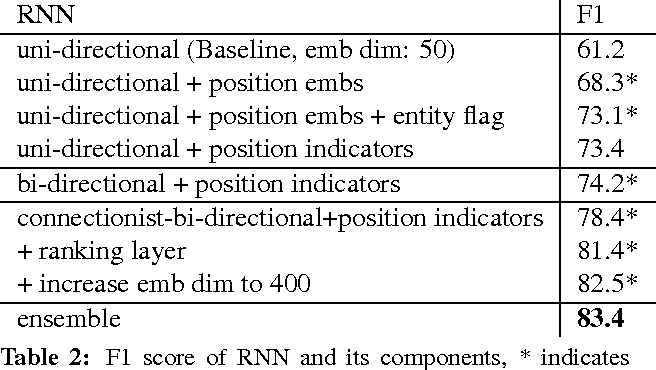This paper investigates two different neural architectures for the task of relation classification: convolutional neural networks and recurrent neural networks. For both models, we demonstrate the effect of different architectural choices. We present a new context representation for convolutional neural networks for relation classification (extended middle context). Furthermore, we propose connectionist bi-directional recurrent neural networks and introduce ranking loss for their optimization. Finally, we show that combining convolutional and recurrent neural networks using a simple voting scheme is accurate enough to improve results. Our neural models achieve state-of-the-art results on the SemEval 2010 relation classification task.

* NAACL 2016
Click to Read Paper and Get Code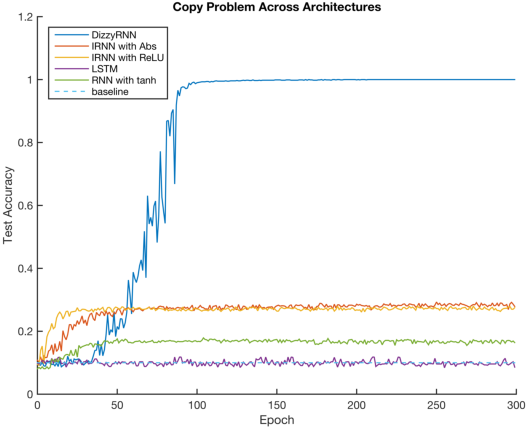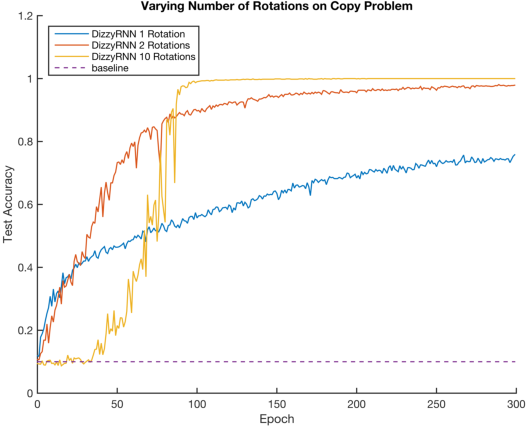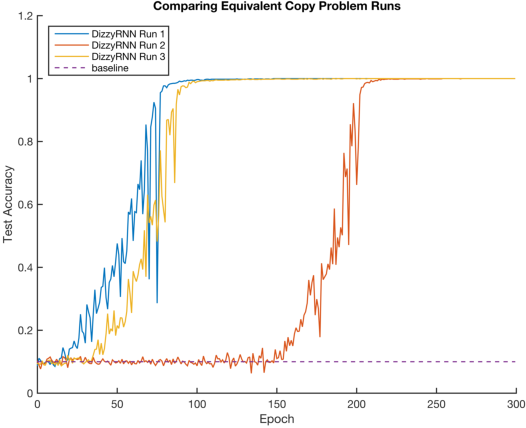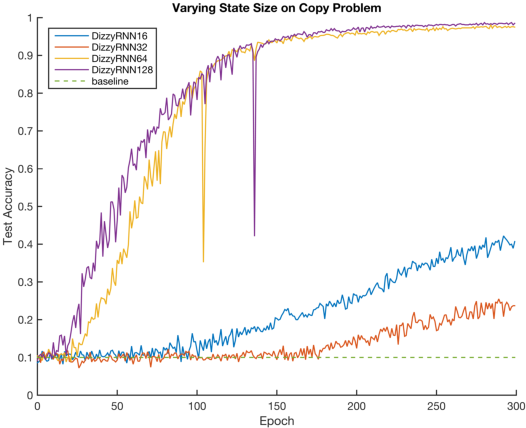The vanishing and exploding gradient problems are well-studied obstacles that make it difficult for recurrent neural networks to learn long-term time dependencies. We propose a reparameterization of standard recurrent neural networks to update linear transformations in a provably norm-preserving way through Givens rotations. Additionally, we use the absolute value function as an element-wise non-linearity to preserve the norm of backpropagated signals over the entire network. We show that this reparameterization reduces the number of parameters and maintains the same algorithmic complexity as a standard recurrent neural network, while outperforming standard recurrent neural networks with orthogonal initializations and Long Short-Term Memory networks on the copy problem.

Click to Read Paper and Get Code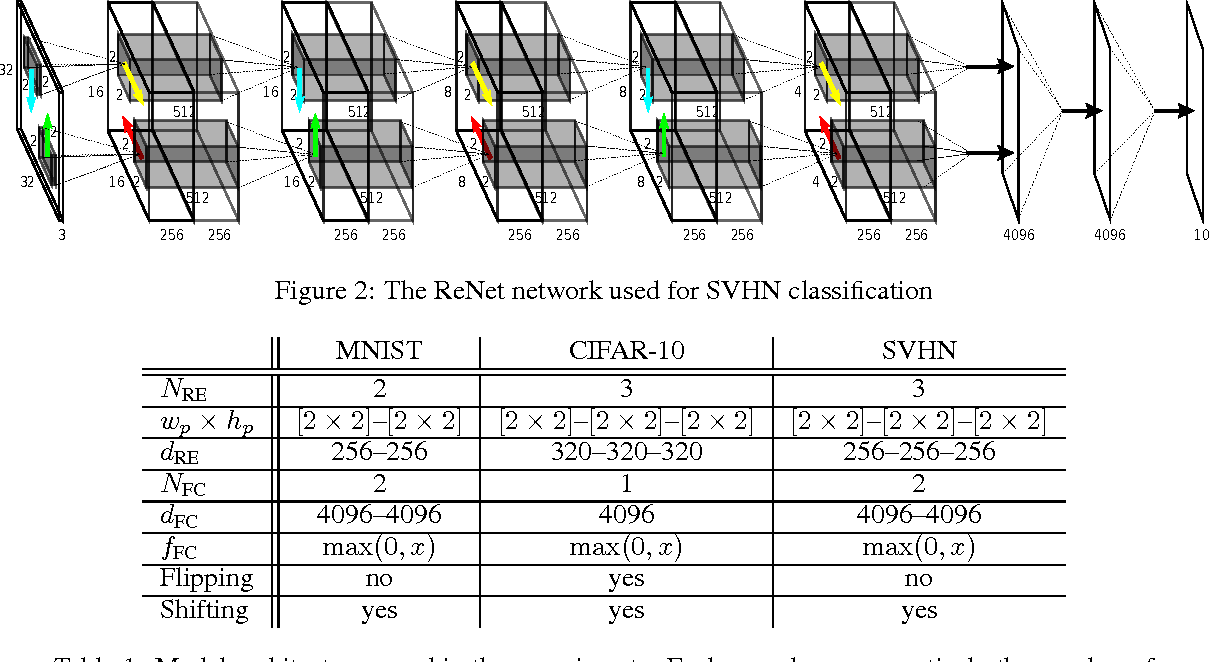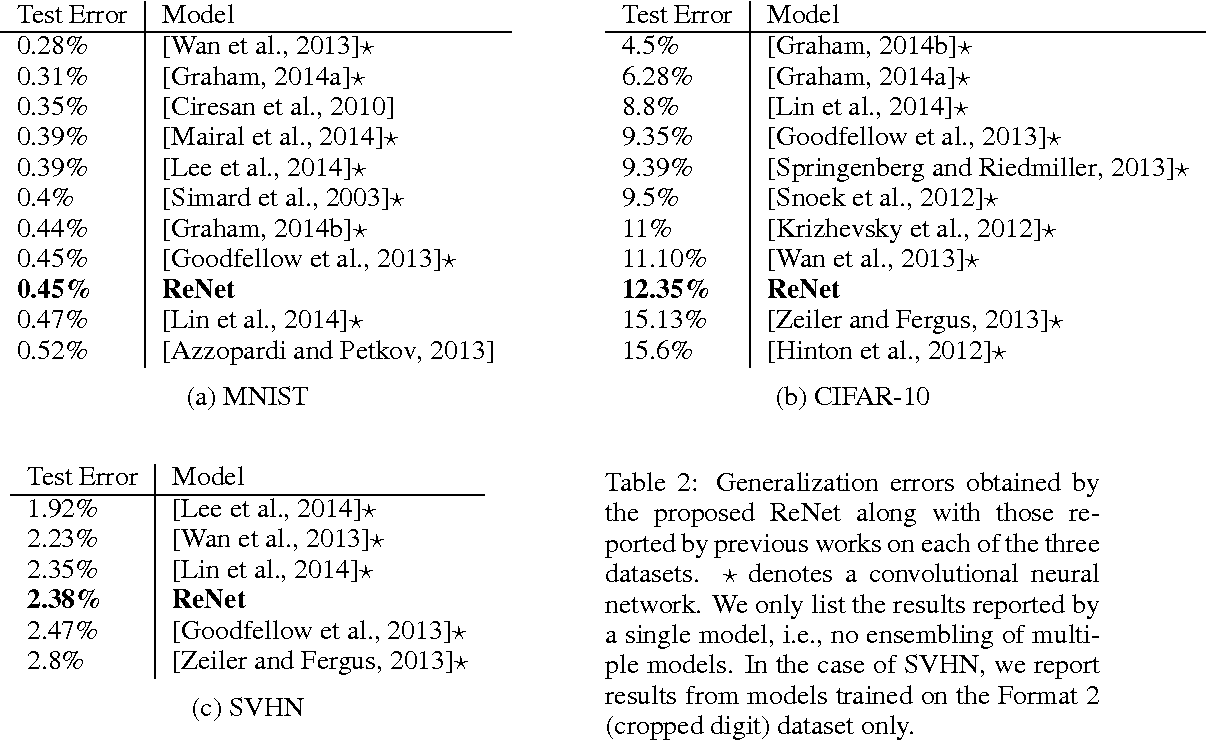In this paper, we propose a deep neural network architecture for object recognition based on recurrent neural networks. The proposed network, called ReNet, replaces the ubiquitous convolution+pooling layer of the deep convolutional neural network with four recurrent neural networks that sweep horizontally and vertically in both directions across the image. We evaluate the proposed ReNet on three widely-used benchmark datasets; MNIST, CIFAR-10 and SVHN. The result suggests that ReNet is a viable alternative to the deep convolutional neural network, and that further investigation is needed.

Click to Read Paper and Get Code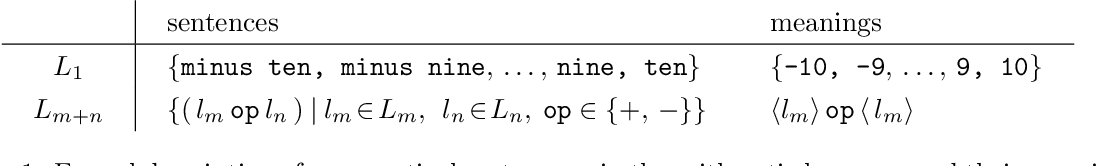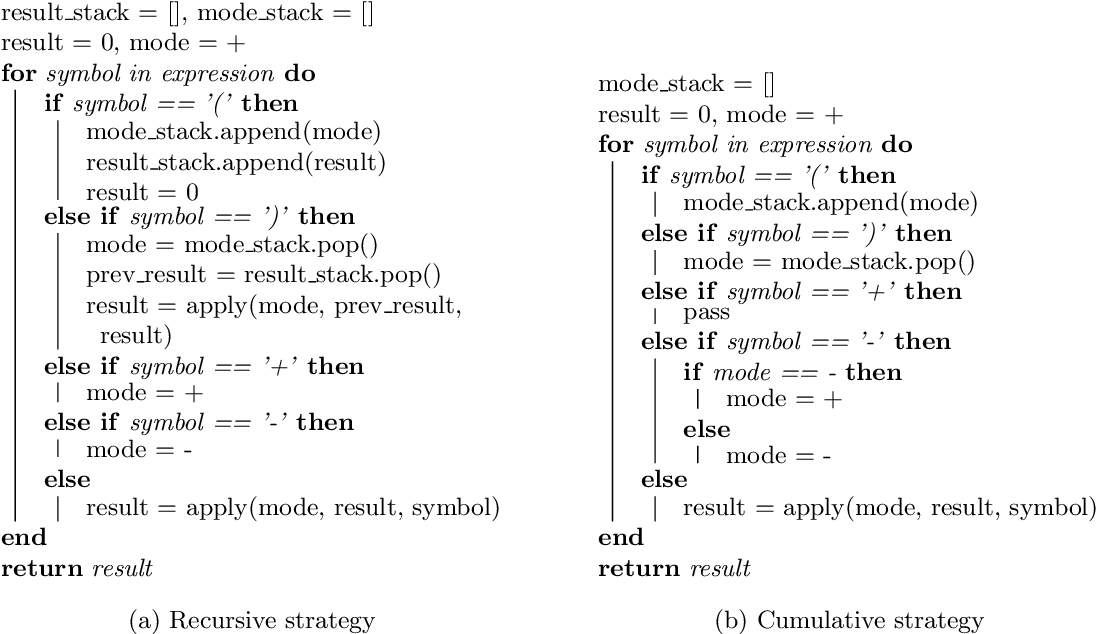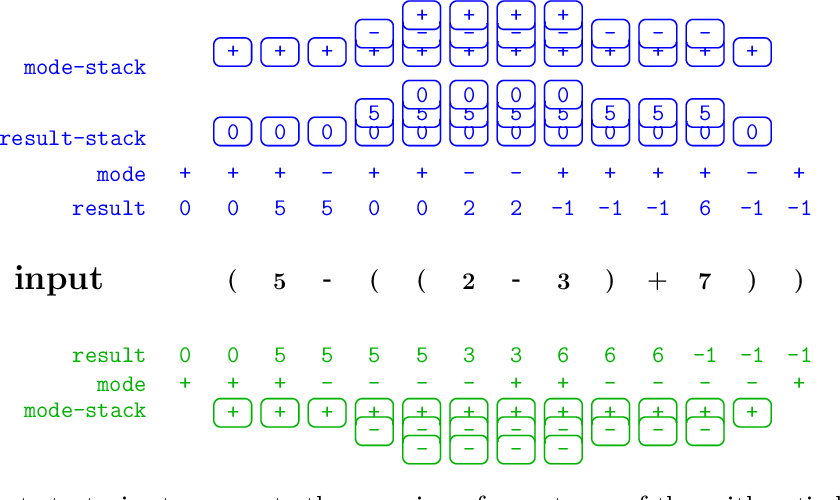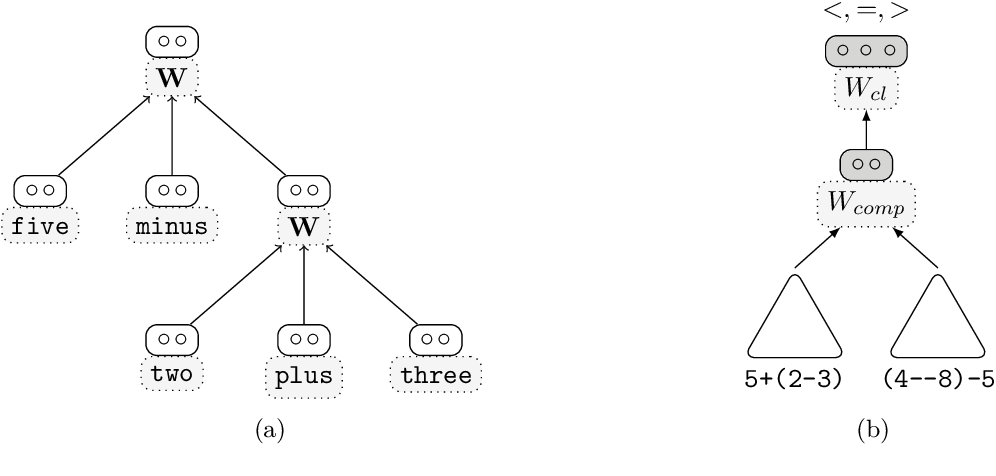We investigate how neural networks can learn and process languages with hierarchical, compositional semantics. To this end, we define the artificial task of processing nested arithmetic expressions, and study whether different types of neural networks can learn to compute their meaning. We find that recursive neural networks can find a generalising solution to this problem, and we visualise this solution by breaking it up in three steps: project, sum and squash. As a next step, we investigate recurrent neural networks, and show that a gated recurrent unit, that processes its input incrementally, also performs very well on this task. To develop an understanding of what the recurrent network encodes, visualisation techniques alone do not suffice. Therefore, we develop an approach where we formulate and test multiple hypotheses on the information encoded and processed by the network. For each hypothesis, we derive predictions about features of the hidden state representations at each time step, and train 'diagnostic classifiers' to test those predictions. Our results indicate that the networks follow a strategy similar to our hypothesised 'cumulative strategy', which explains the high accuracy of the network on novel expressions, the generalisation to longer expressions than seen in training, and the mild deterioration with increasing length. This is turn shows that diagnostic classifiers can be a useful technique for opening up the black box of neural networks. We argue that diagnostic classification, unlike most visualisation techniques, does scale up from small networks in a toy domain, to larger and deeper recurrent networks dealing with real-life data, and may therefore contribute to a better understanding of the internal dynamics of current state-of-the-art models in natural language processing.

* Journal of Artificial Intelligence Research 61 (2018) 907-926
* 20 pages
Click to Read Paper and Get Code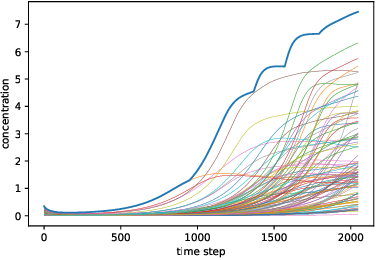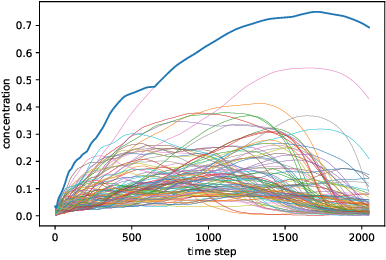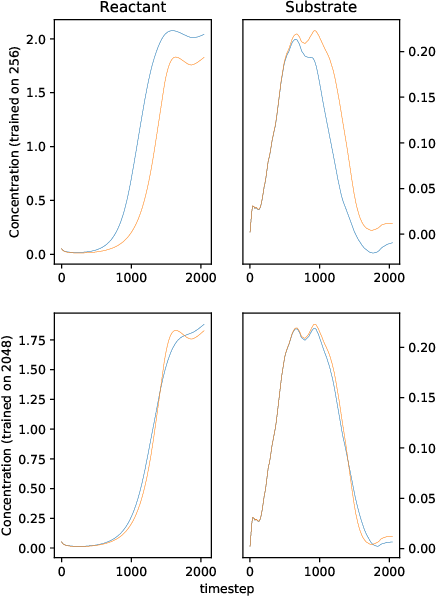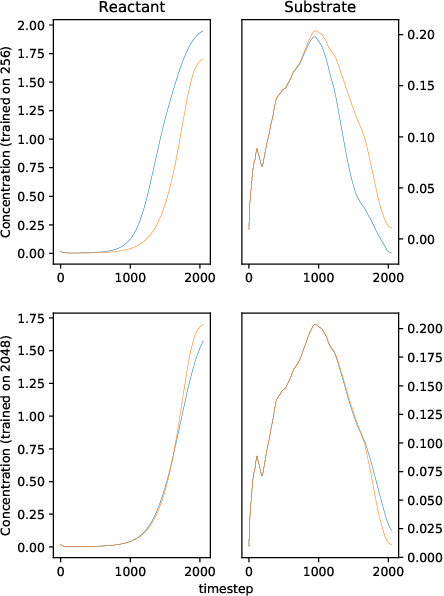We solve a system of ordinary differential equations with an unknown functional form of a sink (reaction rate) term. We assume that the measurements (time series) of state variables are partially available, and we use recurrent neural network to "learn" the reaction rate from this data. This is achieved by including a discretized ordinary differential equations as part of a recurrent neural network training problem. We extend TensorFlow's recurrent neural network architecture to create a simple but scalable and effective solver for the unknown functions, and apply it to a fedbatch bioreactor simulation problem. Use of techniques from recent deep learning literature enables training of functions with behavior manifesting over thousands of time steps. Our networks are structurally similar to recurrent neural networks, but differences in design and function require modifications to the conventional wisdom about training such networks.

* 19 pages, 8 figures
Click to Read Paper and Get Code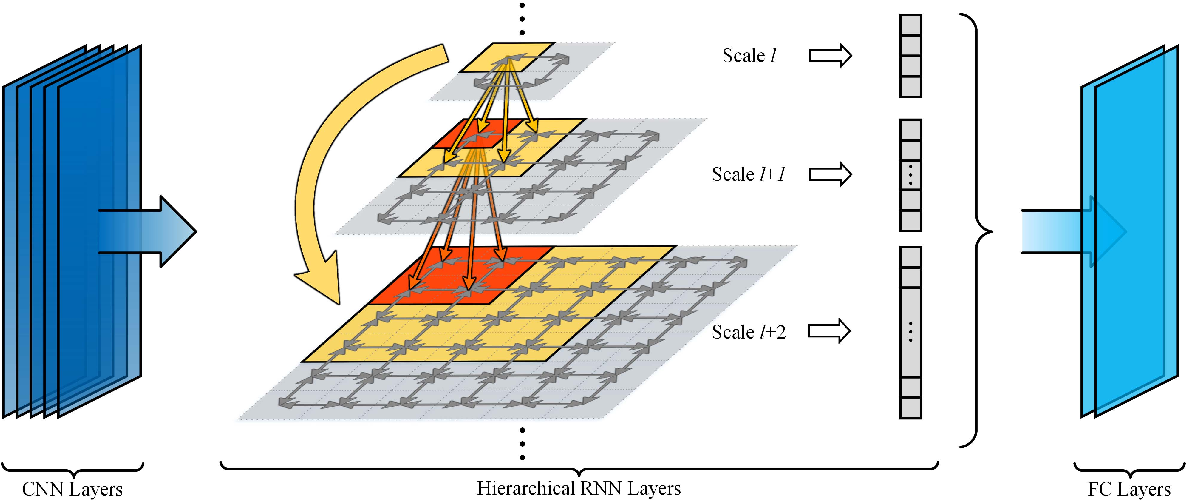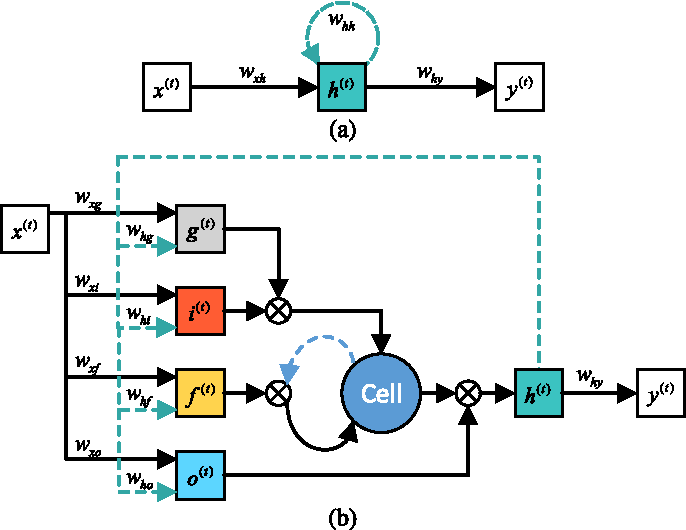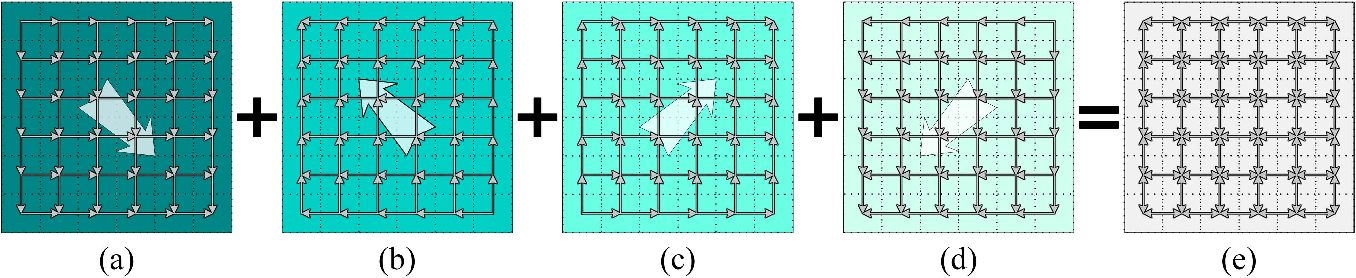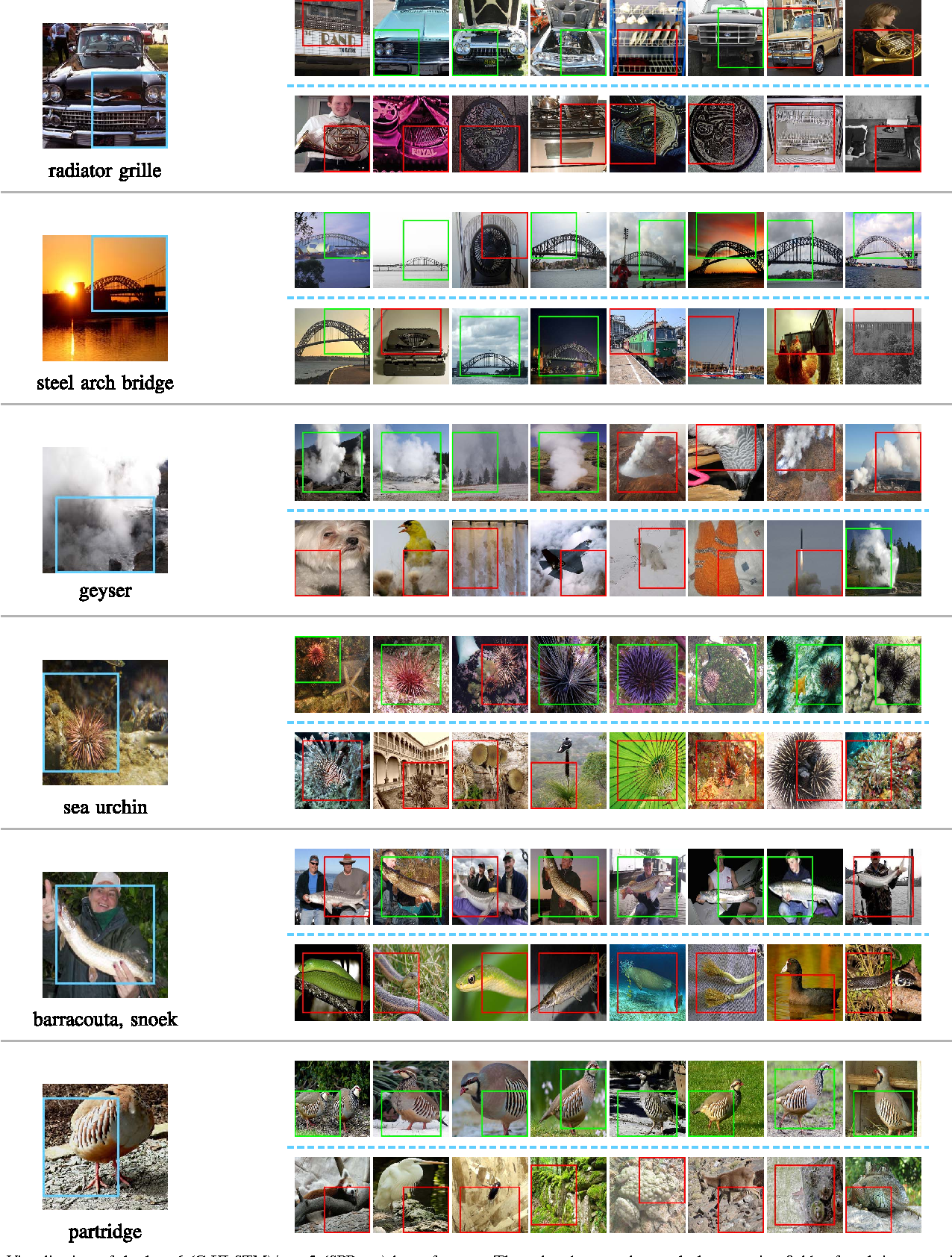Existing deep convolutional neural networks (CNNs) have shown their great success on image classification. CNNs mainly consist of convolutional and pooling layers, both of which are performed on local image areas without considering the dependencies among different image regions. However, such dependencies are very important for generating explicit image representation. In contrast, recurrent neural networks (RNNs) are well known for their ability of encoding contextual information among sequential data, and they only require a limited number of network parameters. General RNNs can hardly be directly applied on non-sequential data. Thus, we proposed the hierarchical RNNs (HRNNs). In HRNNs, each RNN layer focuses on modeling spatial dependencies among image regions from the same scale but different locations. While the cross RNN scale connections target on modeling scale dependencies among regions from the same location but different scales. Specifically, we propose two recurrent neural network models: 1) hierarchical simple recurrent network (HSRN), which is fast and has low computational cost; and 2) hierarchical long-short term memory recurrent network (HLSTM), which performs better than HSRN with the price of more computational cost. In this manuscript, we integrate CNNs with HRNNs, and develop end-to-end convolutional hierarchical recurrent neural networks (C-HRNNs). C-HRNNs not only make use of the representation power of CNNs, but also efficiently encodes spatial and scale dependencies among different image regions. On four of the most challenging object/scene image classification benchmarks, our C-HRNNs achieve state-of-the-art results on Places 205, SUN 397, MIT indoor, and competitive results on ILSVRC 2012.

Click to Read Paper and Get Code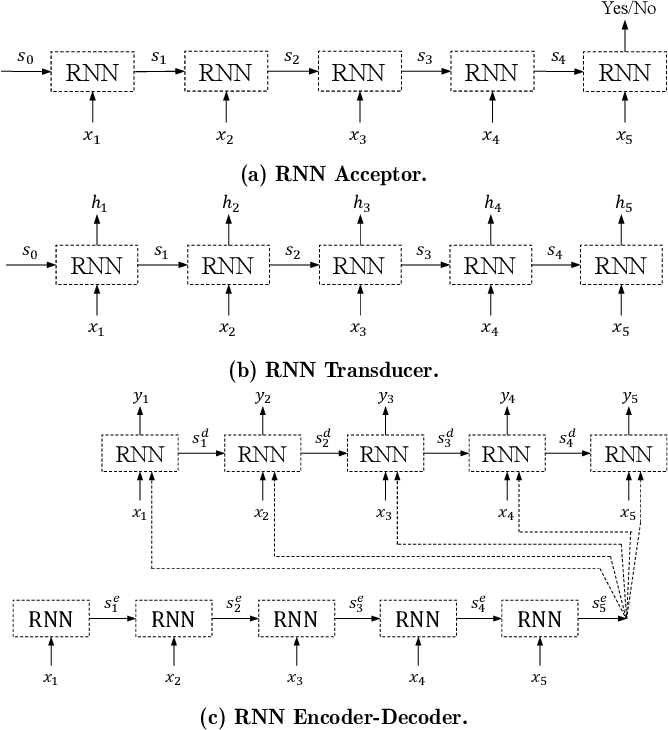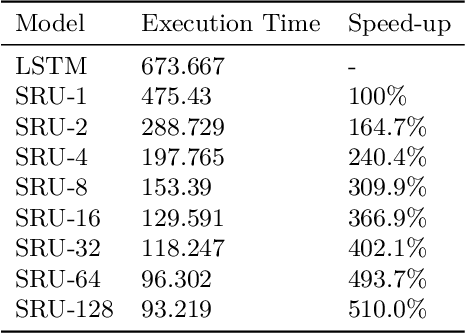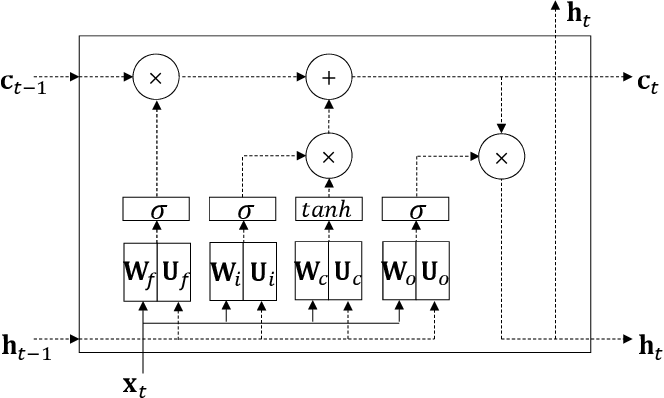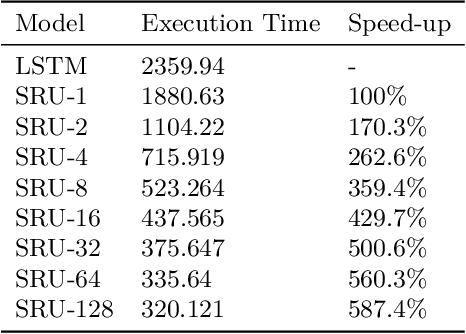As neural network algorithms show high performance in many applications, their efficient inference on mobile and embedded systems are of great interests. When a single stream recurrent neural network (RNN) is executed for a personal user in embedded systems, it demands a large amount of DRAM accesses because the network size is usually much bigger than the cache size and the weights of an RNN are used only once at each time step. We overcome this problem by parallelizing the algorithm and executing it multiple time steps at a time. This approach also reduces the power consumption by lowering the number of DRAM accesses. QRNN (Quasi Recurrent Neural Networks) and SRU (Simple Recurrent Unit) based recurrent neural networks are used for implementation. The experiments for SRU showed about 300% and 930% of speed-up when the numbers of multi time steps are 4 and 16, respectively, in an ARM CPU based system.

* Submitted to International Conference on Embedded Computer Systems: Architectures, MOdeling and Simulation (SAMOS) 2018
Click to Read Paper and Get Code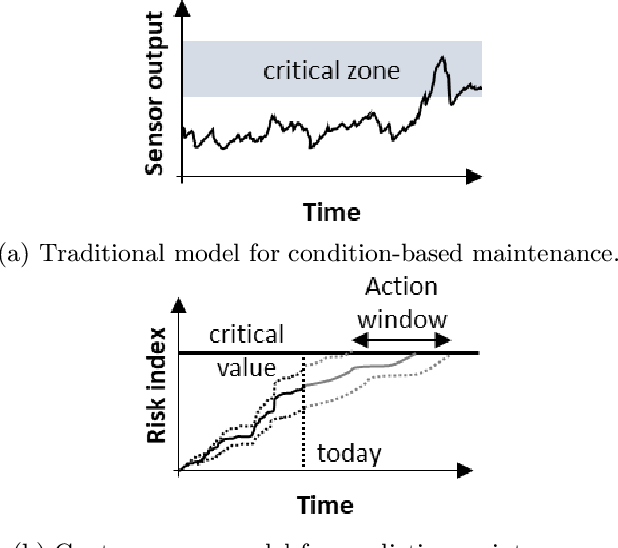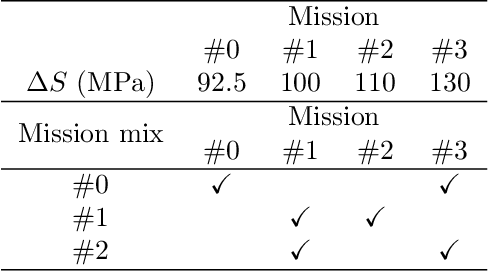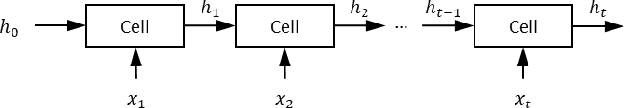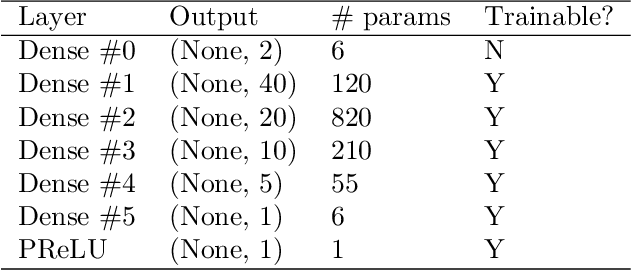Services and warranties of large fleets of engineering assets is a very profitable business. The success of companies in that area is often related to predictive maintenance driven by advanced analytics. Therefore, accurate modeling, as a way to understand how the complex interactions between operating conditions and component capability define useful life, is key for services profitability. Unfortunately, building prognosis models for large fleets is a daunting task as factors such as duty cycle variation, harsh environments, inadequate maintenance, and problems with mass production can lead to large discrepancies between designed and observed useful lives. This paper introduces a novel physics-informed neural network approach to prognosis by extending recurrent neural networks to cumulative damage models. We propose a new recurrent neural network cell designed to merge physics-informed and data-driven layers. With that, engineers and scientists have the chance to use physics-informed layers to model parts that are well understood (e.g., fatigue crack growth) and use data-driven layers to model parts that are poorly characterized (e.g., internal loads). A simple numerical experiment is used to present the main features of the proposed physics-informed recurrent neural network for damage accumulation. The test problem consist of predicting fatigue crack length for a synthetic fleet of airplanes subject to different mission mixes. The model is trained using full observation inputs (far-field loads) and very limited observation of outputs (crack length at inspection for only a portion of the fleet). The results demonstrate that our proposed hybrid physics-informed recurrent neural network is able to accurately model fatigue crack growth even when the observed distribution of crack length does not match with the (unobservable) fleet distribution.

* Data and codes (including our implementation for both the multi-layer perceptron, the stress intensity and Paris law layers, the cumulative damage cell, as well as python driver scripts) used in this manuscript are publicly available on GitHub at https://github.com/PML-UCF/pinn. The data and code are released under the MIT License
Click to Read Paper and Get Code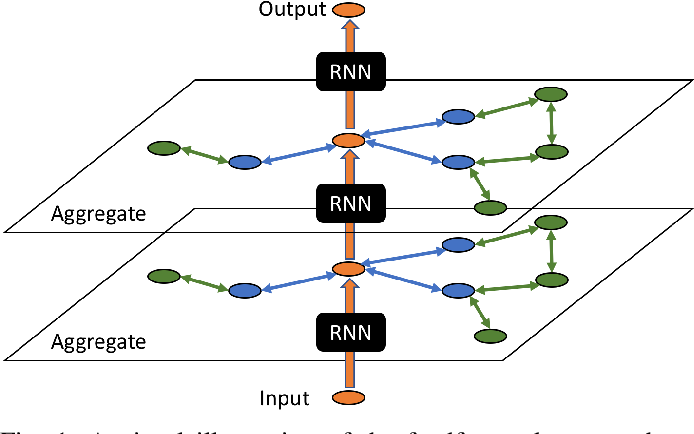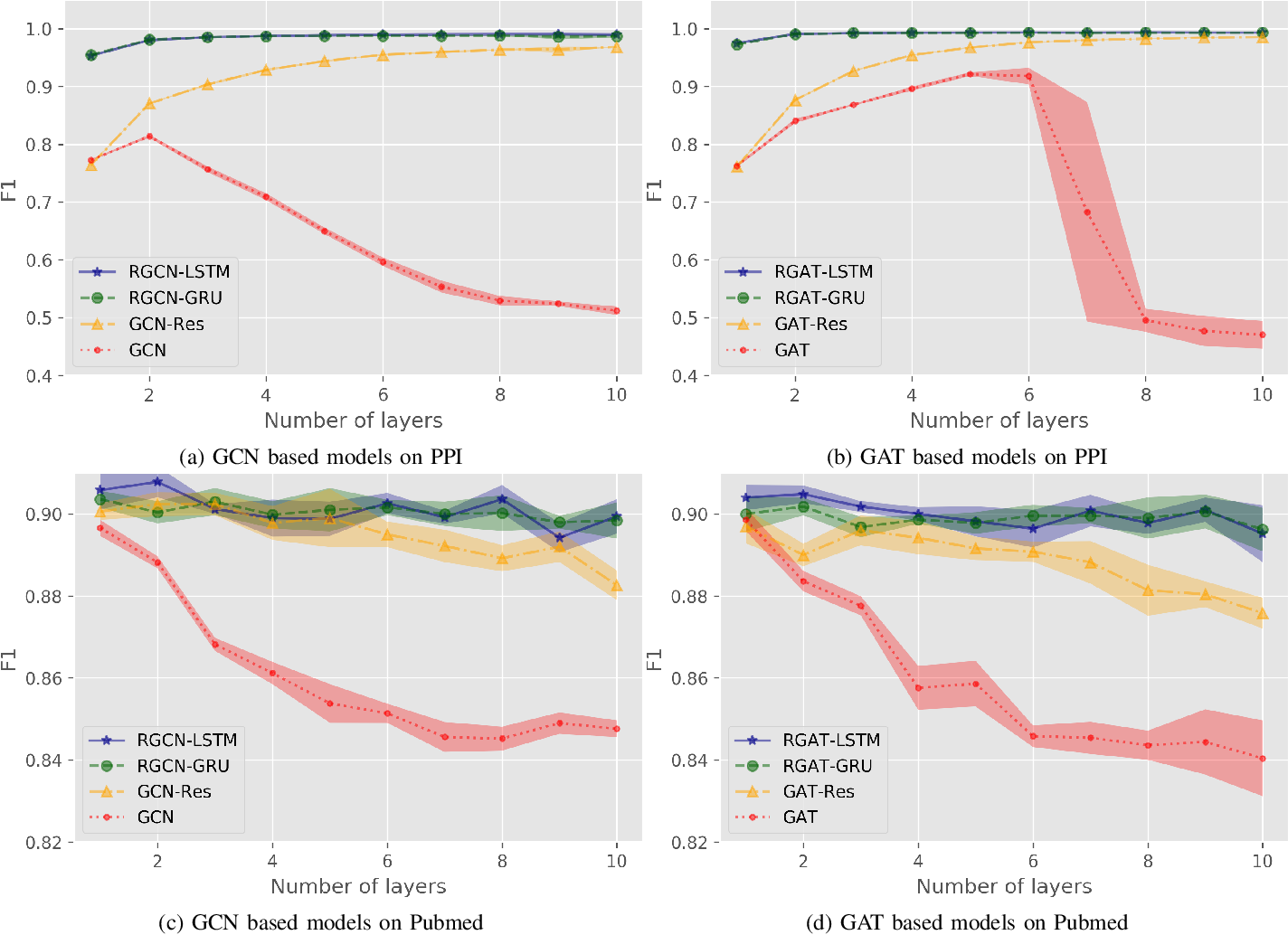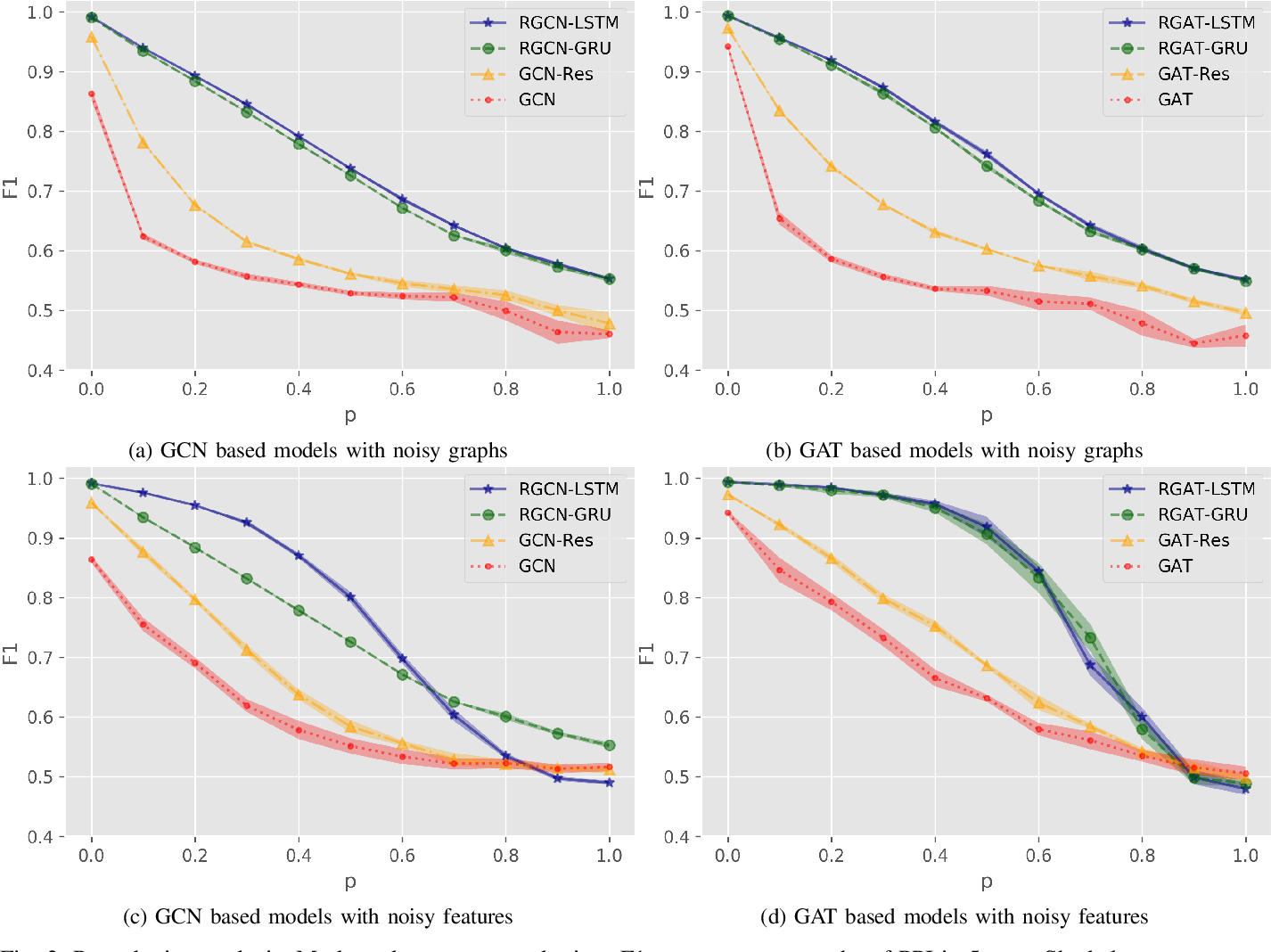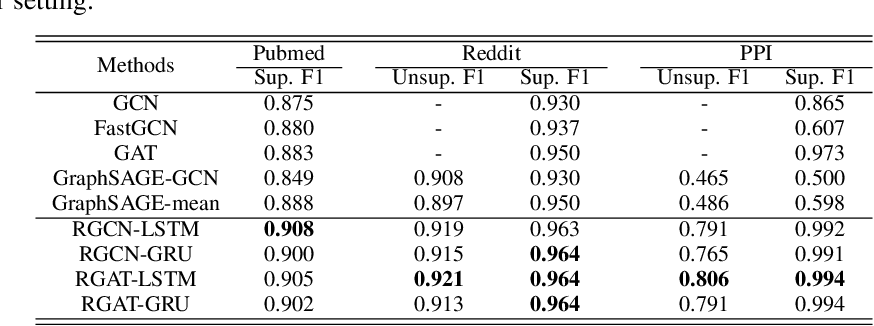In this paper, we study the problem of node representation learning with graph neural networks. We present a graph neural network class named recurrent graph neural network (RGNN), that address the shortcomings of prior methods. By using recurrent units to capture the long-term dependency across layers, our methods can successfully identify important information during recursive neighborhood expansion. In our experiments, we show that our model class achieves state-of-the-art results on three benchmarks: the Pubmed, Reddit, and PPI network datasets. Our in-depth analyses also demonstrate that incorporating recurrent units is a simple yet effective method to prevent noisy information in graphs, which enables a deeper graph neural network.

Click to Read Paper and Get Code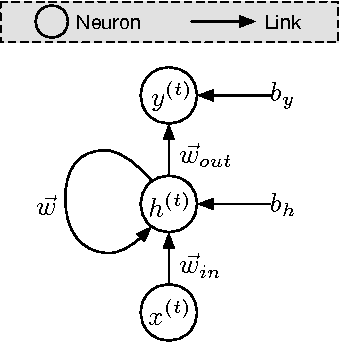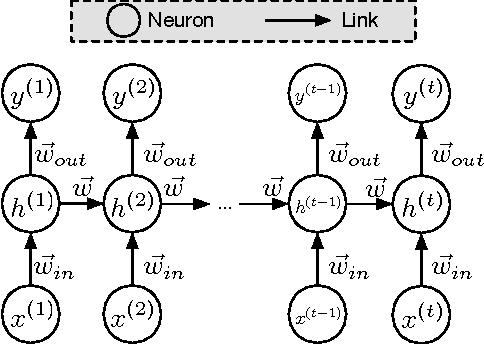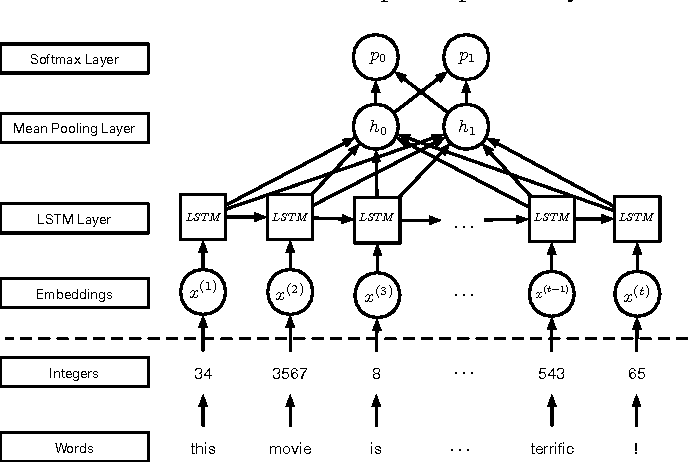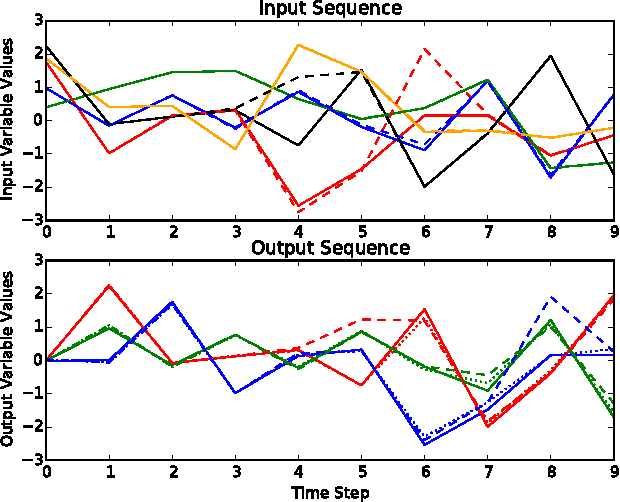Machine learning models are frequently used to solve complex security problems, as well as to make decisions in sensitive situations like guiding autonomous vehicles or predicting financial market behaviors. Previous efforts have shown that numerous machine learning models were vulnerable to adversarial manipulations of their inputs taking the form of adversarial samples. Such inputs are crafted by adding carefully selected perturbations to legitimate inputs so as to force the machine learning model to misbehave, for instance by outputting a wrong class if the machine learning task of interest is classification. In fact, to the best of our knowledge, all previous work on adversarial samples crafting for neural network considered models used to solve classification tasks, most frequently in computer vision applications. In this paper, we contribute to the field of adversarial machine learning by investigating adversarial input sequences for recurrent neural networks processing sequential data. We show that the classes of algorithms introduced previously to craft adversarial samples misclassified by feed-forward neural networks can be adapted to recurrent neural networks. In a experiment, we show that adversaries can craft adversarial sequences misleading both categorical and sequential recurrent neural networks.

Click to Read Paper and Get Code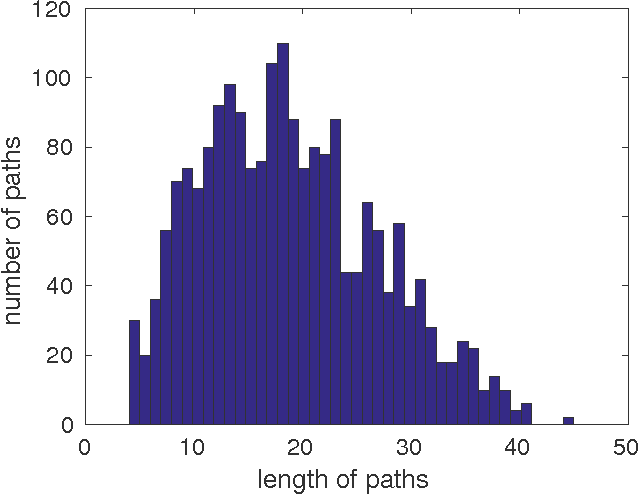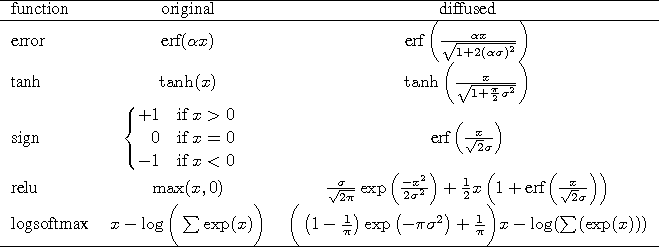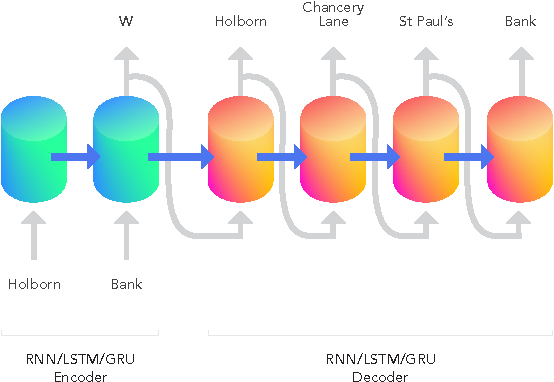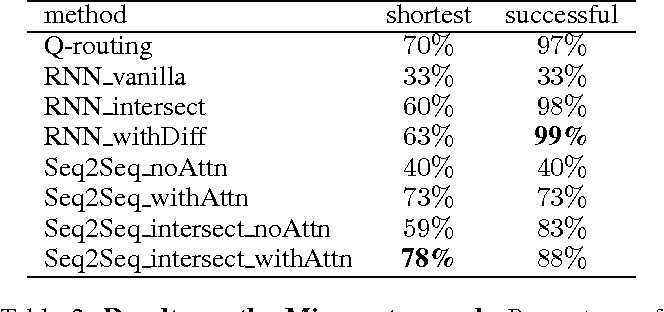Much combinatorial optimisation problems constitute a non-polynomial (NP) hard optimisation problem, i.e., they can not be solved in polynomial time. One such problem is finding the shortest route between two nodes on a graph. Meta-heuristic algorithms such as $A^{*}$ along with mixed-integer programming (MIP) methods are often employed for these problems. Our work demonstrates that it is possible to approximate solutions generated by a meta-heuristic algorithm using a deep recurrent neural network. We compare different methodologies based on reinforcement learning (RL) and recurrent neural networks (RNN) to gauge their respective quality of approximation. We show the viability of recurrent neural network solutions on a graph that has over 300 nodes and argue that a sequence-to-sequence network rather than other recurrent networks has improved approximation quality. Additionally, we argue that homotopy continuation -- that increases chances of hitting an extremum -- further improves the estimate generated by a vanilla RNN.

Click to Read Paper and Get Code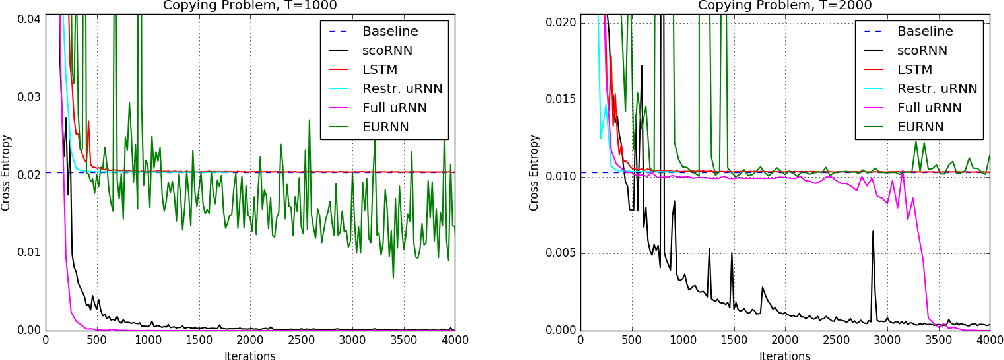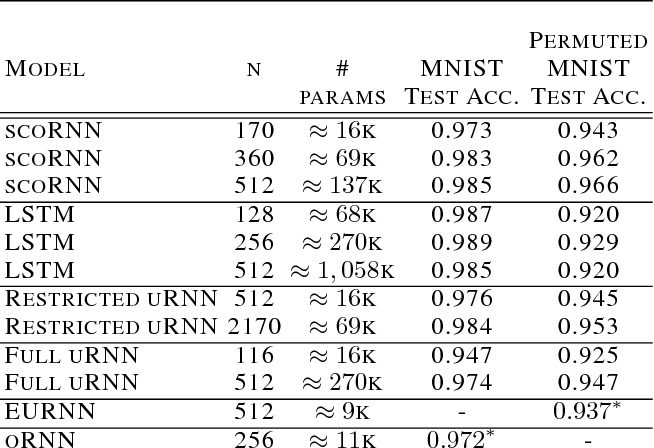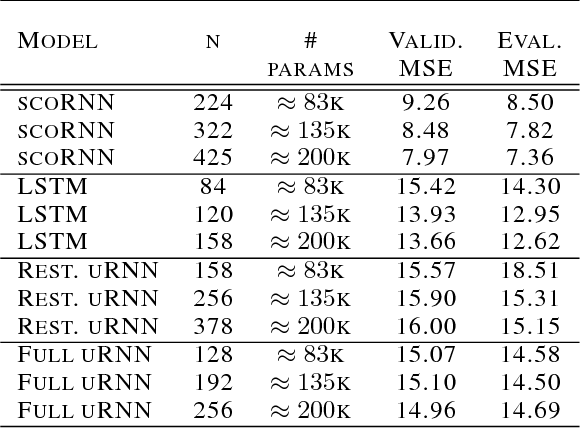Recurrent Neural Networks (RNNs) are designed to handle sequential data but suffer from vanishing or exploding gradients. Recent work on Unitary Recurrent Neural Networks (uRNNs) have been used to address this issue and in some cases, exceed the capabilities of Long Short-Term Memory networks (LSTMs). We propose a simpler and novel update scheme to maintain orthogonal recurrent weight matrices without using complex valued matrices. This is done by parametrizing with a skew-symmetric matrix using the Cayley transform. Such a parametrization is unable to represent matrices with negative one eigenvalues, but this limitation is overcome by scaling the recurrent weight matrix by a diagonal matrix consisting of ones and negative ones. The proposed training scheme involves a straightforward gradient calculation and update step. In several experiments, the proposed scaled Cayley orthogonal recurrent neural network (scoRNN) achieves superior results with fewer trainable parameters than other unitary RNNs.

* 12 pages
Click to Read Paper and Get Code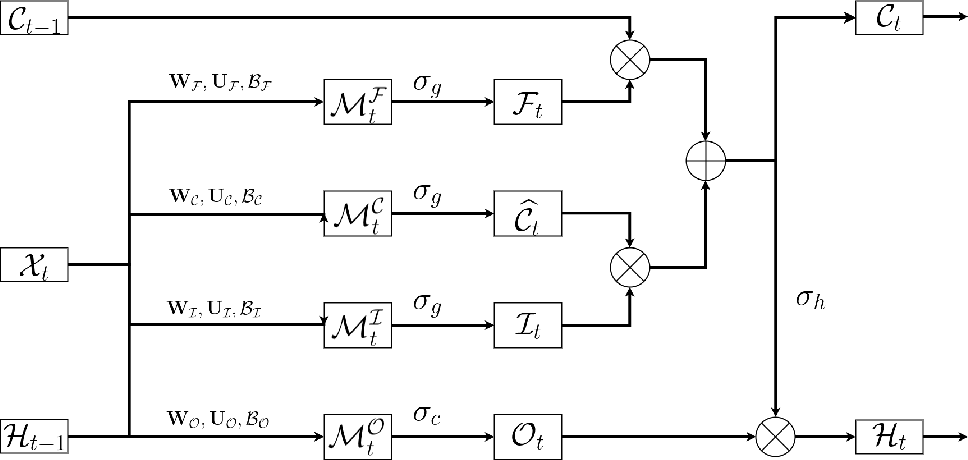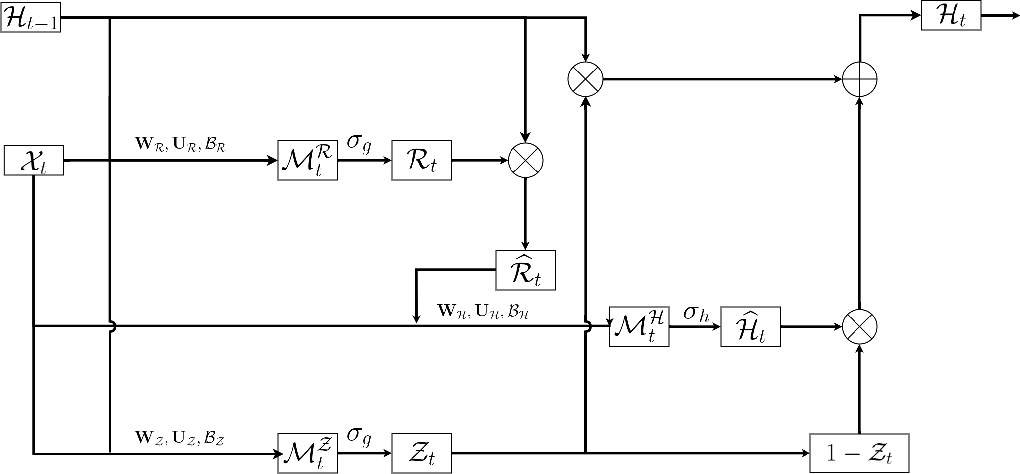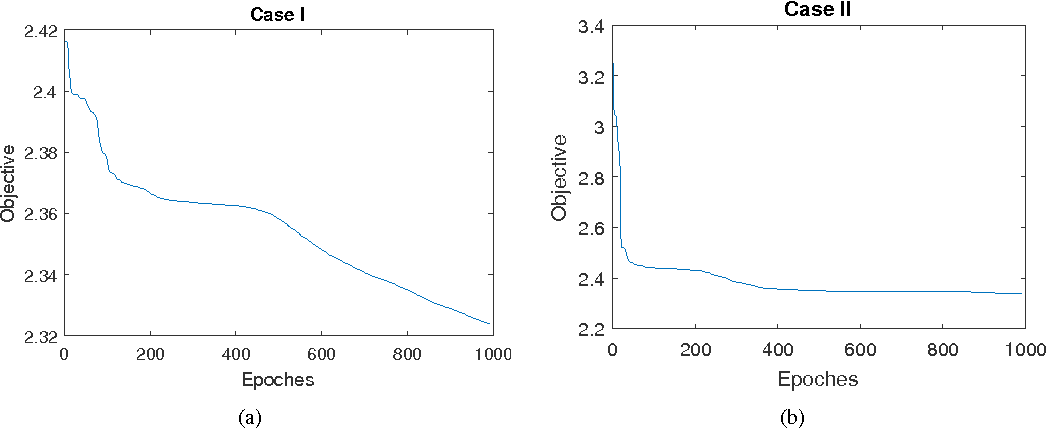Traditional Recurrent Neural Networks assume vectorized data as inputs. However many data from modern science and technology come in certain structures such as tensorial time series data. To apply the recurrent neural networks for this type of data, a vectorisation process is necessary, while such a vectorisation leads to the loss of the precise information of the spatial or longitudinal dimensions. In addition, such a vectorized data is not an optimum solution for learning the representation of the longitudinal data. In this paper, we propose a new variant of tensorial neural networks which directly take tensorial time series data as inputs. We call this new variant as Tensorial Recurrent Neural Network (TRNN). The proposed TRNN is based on tensor Tucker decomposition.

Click to Read Paper and Get Code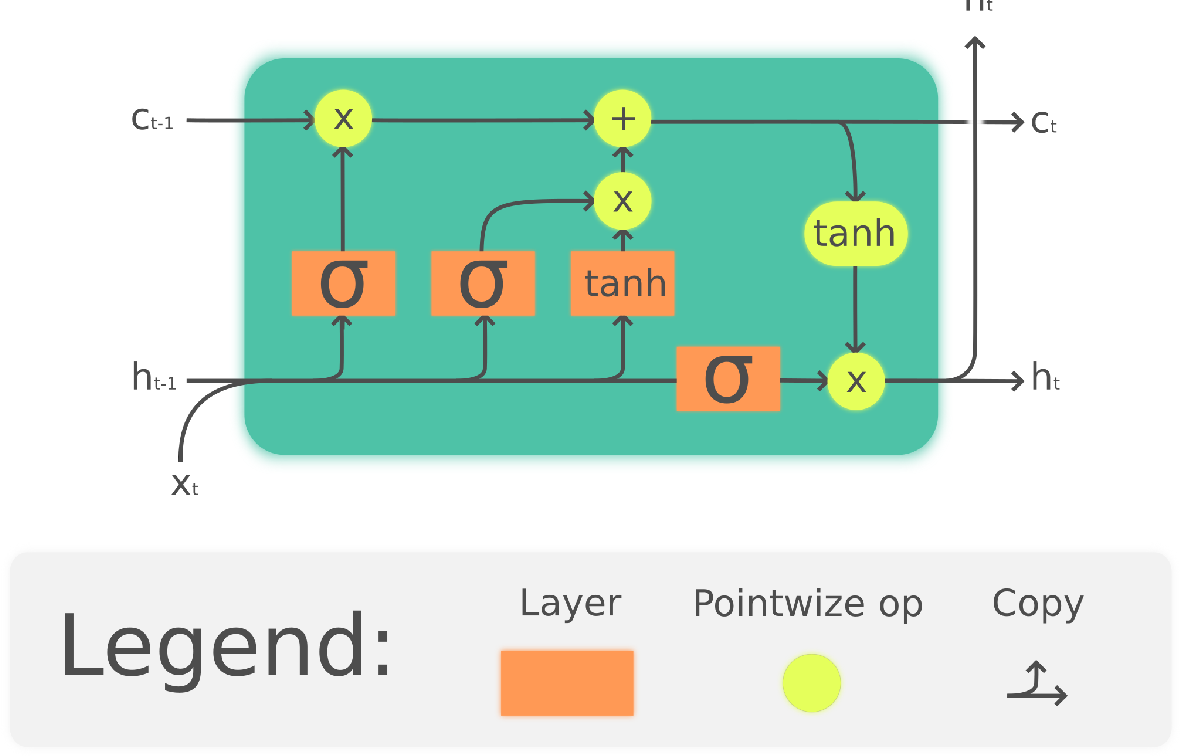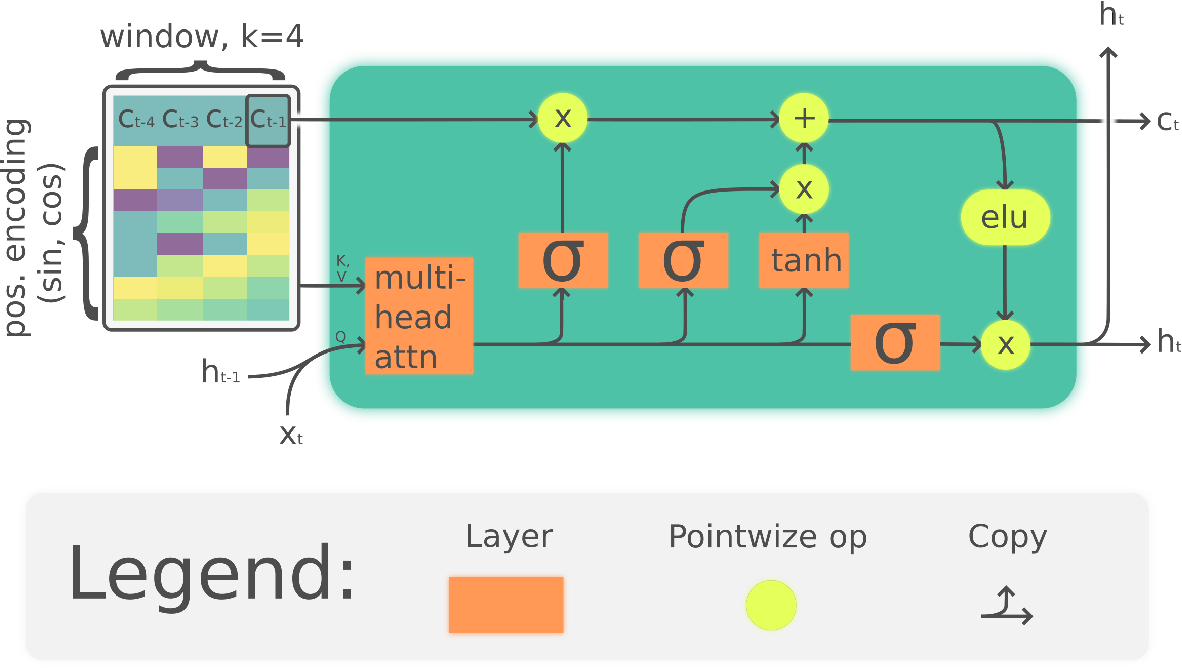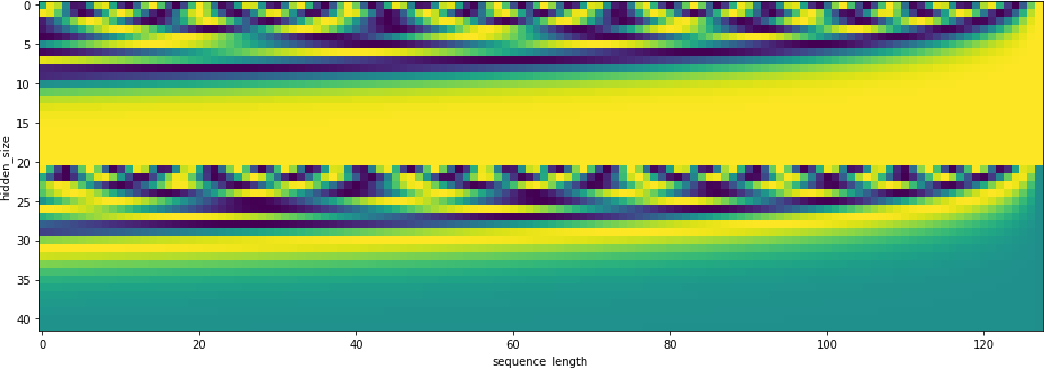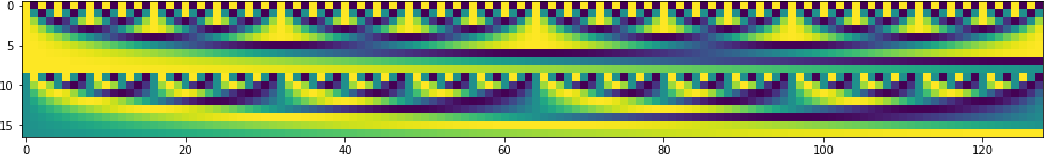The Linear Attention Recurrent Neural Network (LARNN) is a recurrent attention module derived from the Long Short-Term Memory (LSTM) cell and ideas from the consciousness Recurrent Neural Network (RNN). Yes, it LARNNs. The LARNN uses attention on its past cell state values for a limited window size $k$. The formulas are also derived from the Batch Normalized LSTM (BN-LSTM) cell and the Transformer Network for its Multi-Head Attention Mechanism. The Multi-Head Attention Mechanism is used inside the cell such that it can query its own $k$ past values with the attention window. This has the effect of augmenting the rank of the tensor with the attention mechanism, such that the cell can perform complex queries to question its previous inner memories, which should augment the long short-term effect of the memory. With a clever trick, the LARNN cell with attention can be easily used inside a loop on the cell state, just like how any other Recurrent Neural Network (RNN) cell can be looped linearly through time series. This is due to the fact that its state, which is looped upon throughout time steps within time series, stores the inner states in a "first in, first out" queue which contains the $k$ most recent states and on which it is easily possible to add static positional encoding when the queue is represented as a tensor. This neural architecture yields better results than the vanilla LSTM cells. It can obtain results of 91.92% for the test accuracy, compared to the previously attained 91.65% using vanilla LSTM cells. Note that this is not to compare to other research, where up to 93.35% is obtained, but costly using 18 LSTM cells rather than with 2 to 3 cells as analyzed here. Finally, an interesting discovery is made, such that adding activation within the multi-head attention mechanism's linear layers can yield better results in the context researched hereto.

* 14 pages, 10 figures
Click to Read Paper and Get Code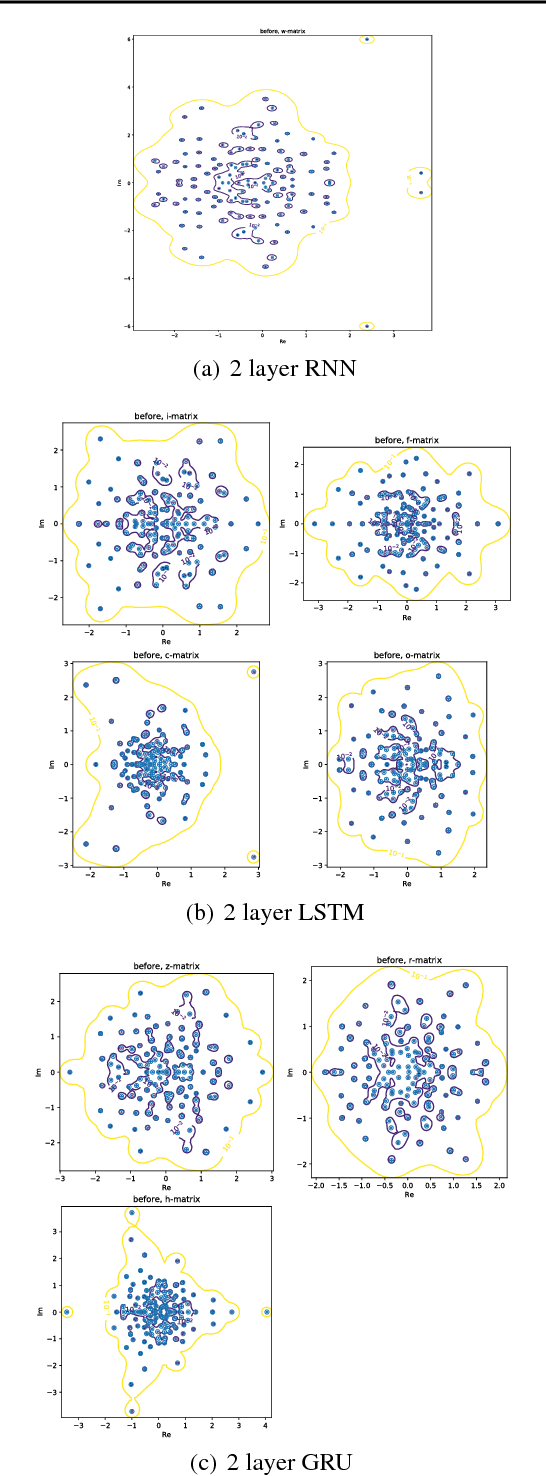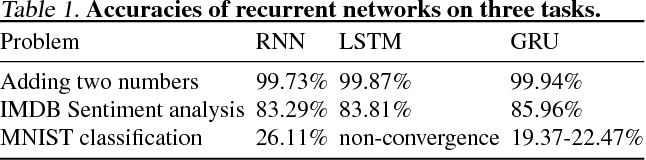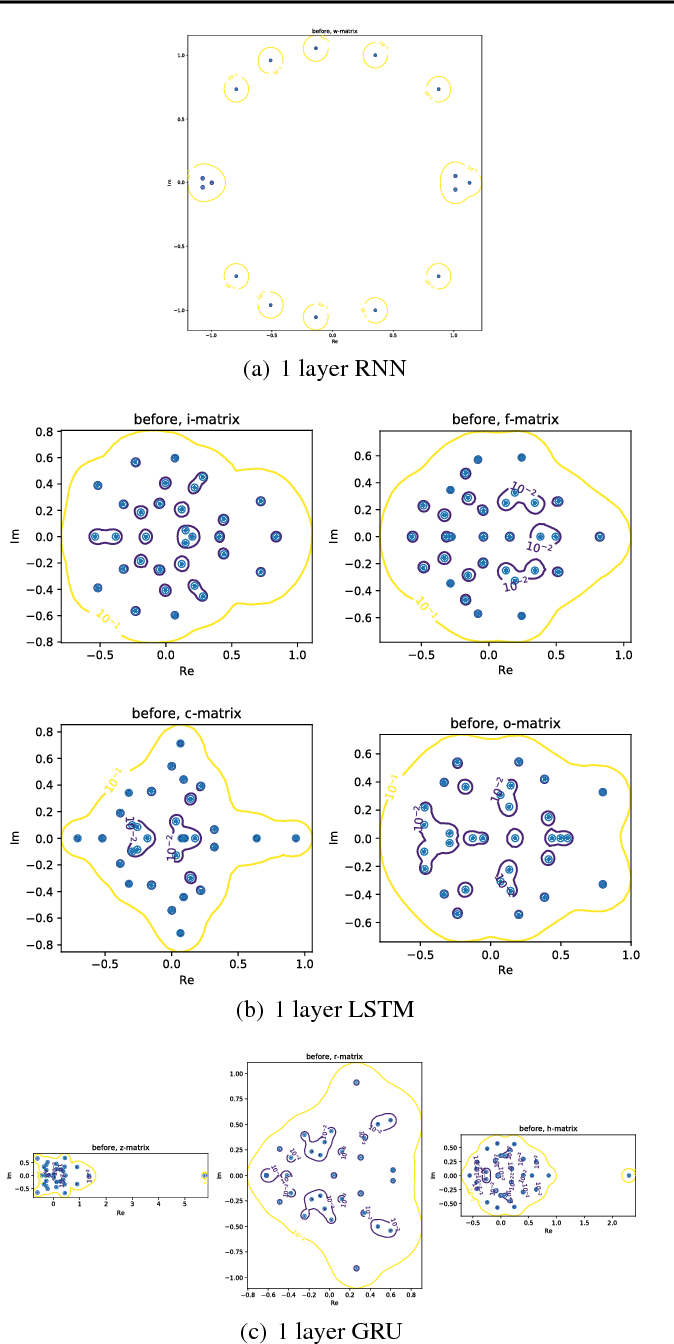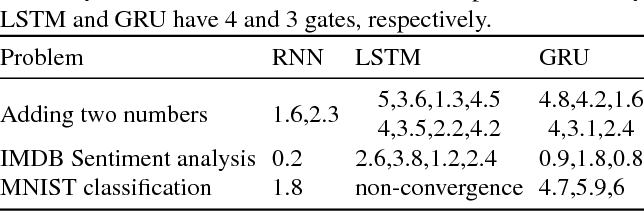Convolutional and Recurrent, deep neural networks have been successful in machine learning systems for computer vision, reinforcement learning, and other allied fields. However, the robustness of such neural networks is seldom apprised, especially after high classification accuracy has been attained. In this paper, we evaluate the robustness of three recurrent neural networks to tiny perturbations, on three widely used datasets, to argue that high accuracy does not always mean a stable and a robust (to bounded perturbations, adversarial attacks, etc.) system. Especially, normalizing the spectrum of the discrete recurrent network to bound the spectrum (using power method, Rayleigh quotient, etc.) on a unit disk produces stable, albeit highly non-robust neural networks. Furthermore, using the $\epsilon$-pseudo-spectrum, we show that training of recurrent networks, say using gradient-based methods, often result in non-normal matrices that may or may not be diagonalizable. Therefore, the open problem lies in constructing methods that optimize not only for accuracy but also for the stability and the robustness of the underlying neural network, a criterion that is distinct from the other.

Click to Read Paper and Get Code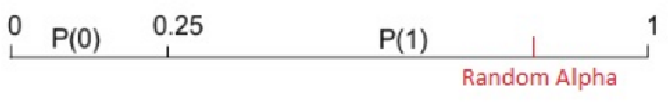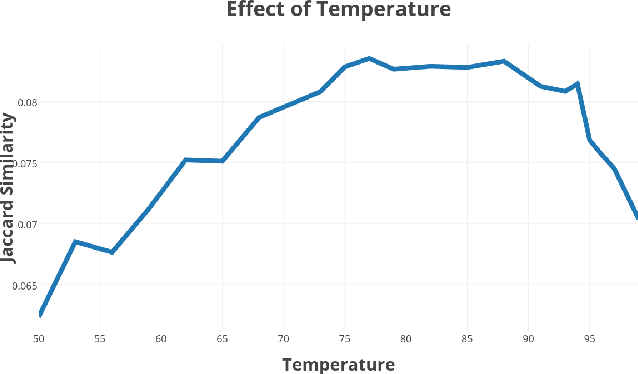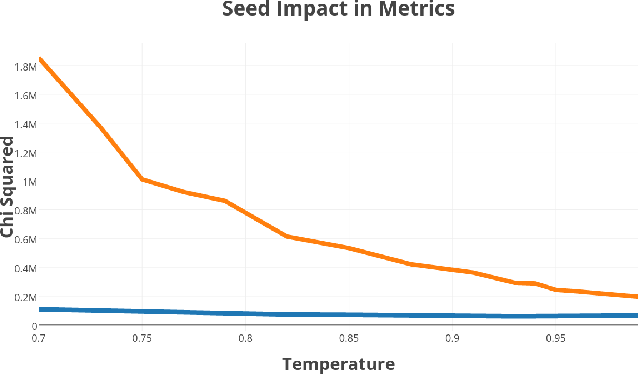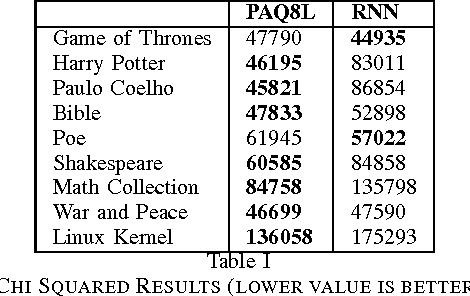In recent studies  Recurrent Neural Networks were used for generative processes and their surprising performance can be explained by their ability to create good predictions. In addition, data compression is also based on predictions. What the problem comes down to is whether a data compressor could be used to perform as well as recurrent neural networks in natural language processing tasks. If this is possible,then the problem comes down to determining if a compression algorithm is even more intelligent than a neural network in specific tasks related to human language. In our journey we discovered what we think is the fundamental difference between a Data Compression Algorithm and a Recurrent Neural Network.

Click to Read Paper and Get Code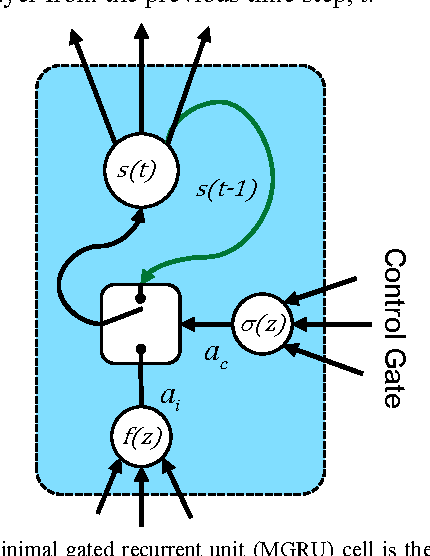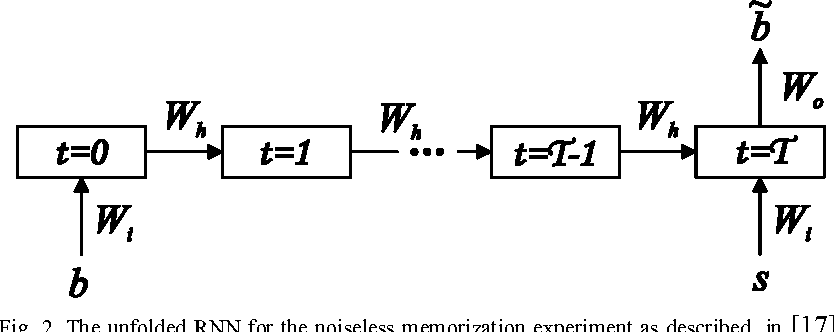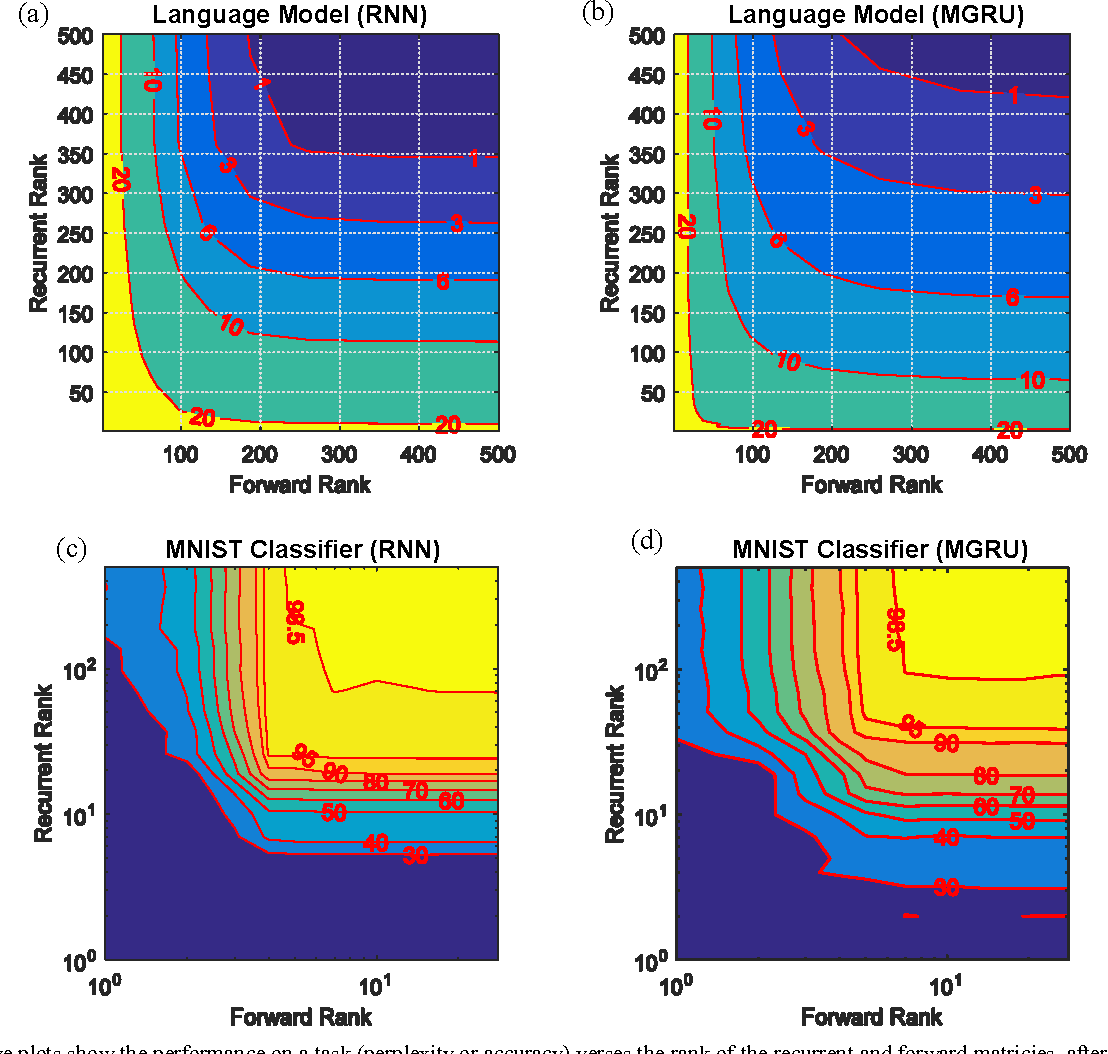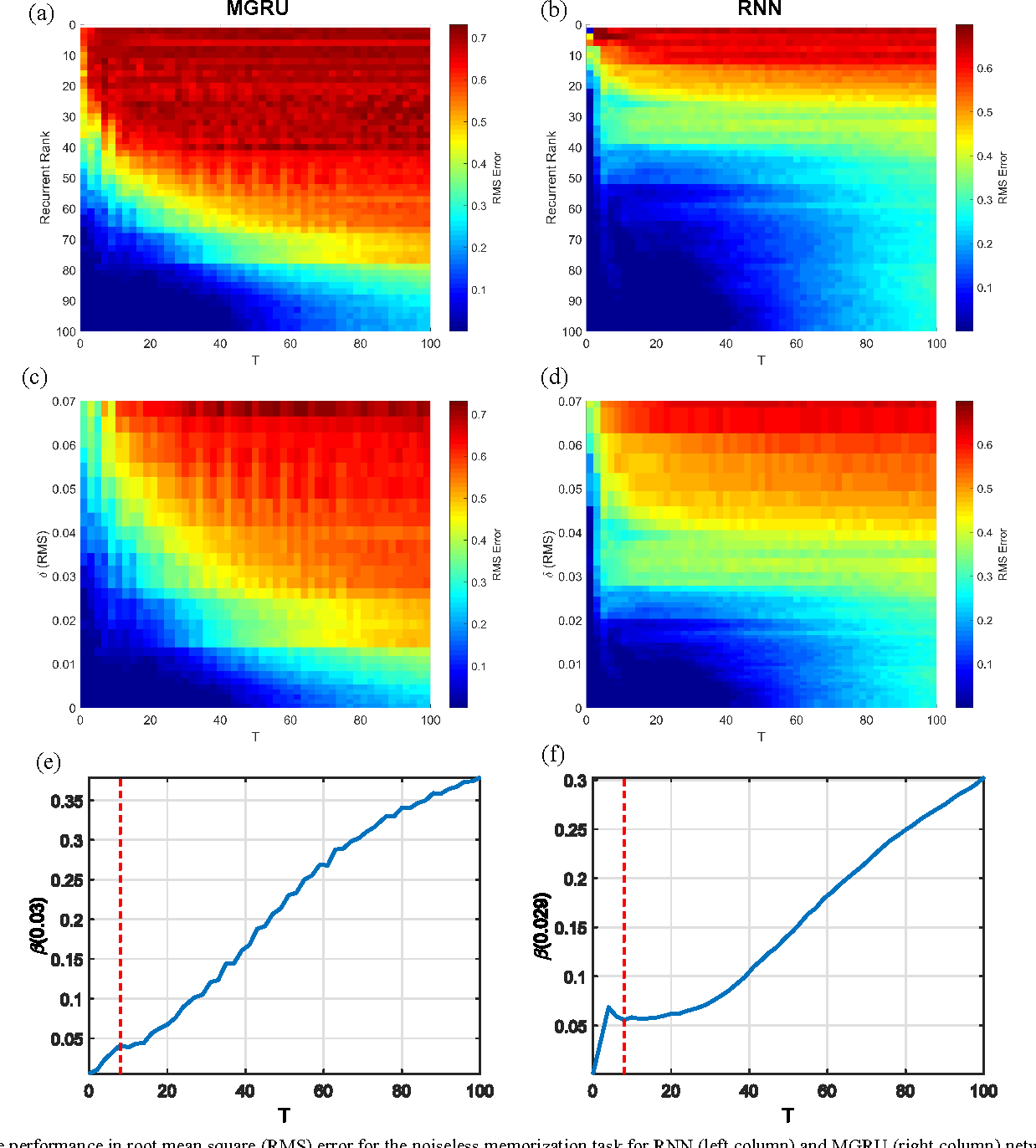The significant computational costs of deploying neural networks in large-scale or resource constrained environments, such as data centers and mobile devices, has spurred interest in model compression, which can achieve a reduction in both arithmetic operations and storage memory. Several techniques have been proposed for reducing or compressing the parameters for feed-forward and convolutional neural networks, but less is understood about the effect of parameter compression on recurrent neural networks (RNN). In particular, the extent to which the recurrent parameters can be compressed and the impact on short-term memory performance, is not well understood. In this paper, we study the effect of complexity reduction, through singular value decomposition rank reduction, on RNN and minimal gated recurrent unit (MGRU) networks for several tasks. We show that considerable rank reduction is possible when compressing recurrent weights, even without fine tuning. Furthermore, we propose a perturbation model for the effect of general perturbations, such as a compression, on the recurrent parameters of RNNs. The model is tested against a noiseless memorization experiment that elucidates the short-term memory performance. In this way, we demonstrate that the effect of compression of recurrent parameters is dependent on the degree of temporal coherence present in the data and task. This work can guide on-the-fly RNN compression for novel environments or tasks, and provides insight for applying RNN compression in low-power devices, such as hearing aids.

* Accepted to IJCNN 2017. Final camera ready paper
Click to Read Paper and Get Code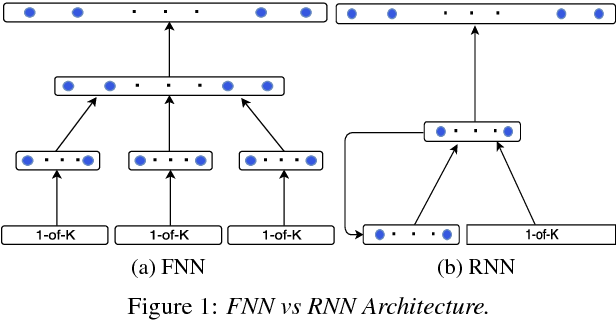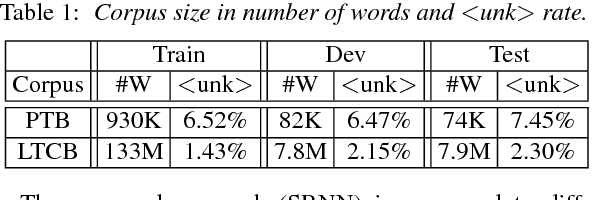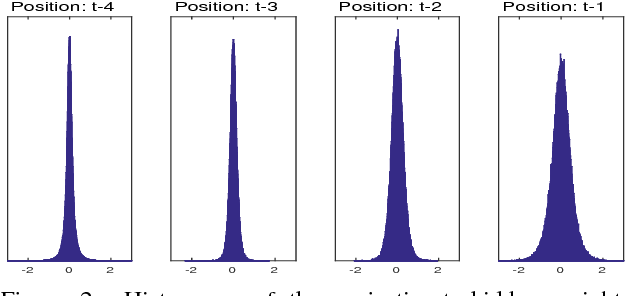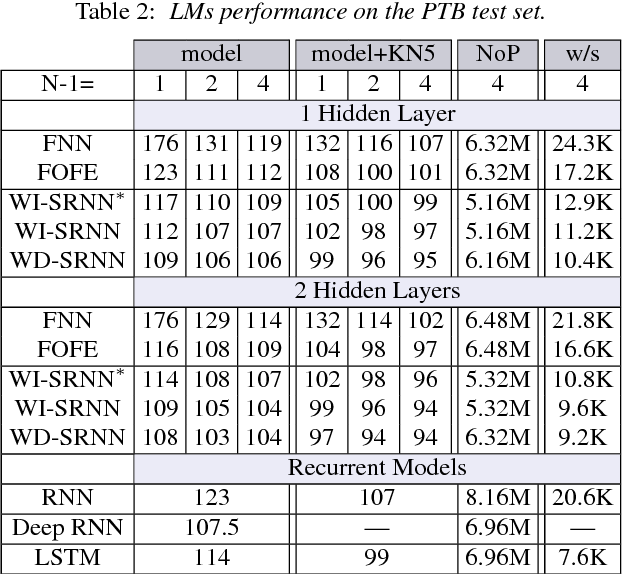Feedforward Neural Network (FNN)-based language models estimate the probability of the next word based on the history of the last N words, whereas Recurrent Neural Networks (RNN) perform the same task based only on the last word and some context information that cycles in the network. This paper presents a novel approach, which bridges the gap between these two categories of networks. In particular, we propose an architecture which takes advantage of the explicit, sequential enumeration of the word history in FNN structure while enhancing each word representation at the projection layer through recurrent context information that evolves in the network. The context integration is performed using an additional word-dependent weight matrix that is also learned during the training. Extensive experiments conducted on the Penn Treebank (PTB) and the Large Text Compression Benchmark (LTCB) corpus showed a significant reduction of the perplexity when compared to state-of-the-art feedforward as well as recurrent neural network architectures.

* published (INTERSPEECH 2016), 5 pages, 3 figures, 4 tables
Click to Read Paper and Get Code